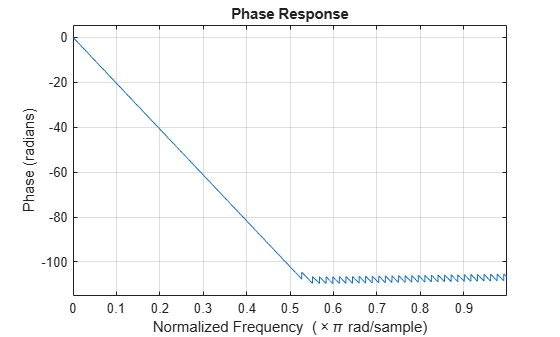# phasez

Unwrapped phase response for filter

## Syntax

``````[phi,w] = phasez(sysobj)``````
``````[phi,w] = phasez(sysobj,n)``````
``````[phi,w] = phasez(sysobj,'Arithmetic',arithType)``````
``phasez(sysobj)``

## Description

``````[phi,w] = phasez(sysobj)``` returns the unwrapped phase response `phi` of the filter System object™, `sysobj`, based on the current filter coefficients. The vector `w` contains the frequencies (in radians) at which the function evaluates the phase response. The phase response is evaluated at 8192 points equally spaced around the upper half of the unit circle.```
``````[phi,w] = phasez(sysobj,n)``` returns the phase response of the filter System object and the corresponding frequencies at `n` points equally spaced around the upper half of the unit circle.```
``````[phi,w] = phasez(sysobj,'Arithmetic',arithType)``` analyzes the filter System object, based on the arithmetic specified in `arithType`, using either of the previous syntaxes.```

example

````phasez(sysobj)` displays the phase response of the filter System object `sysobj` in the `fvtool`.For more input options, see `phasez`.```

## Examples

collapse all

```Fs = 8000; Fcutoff = 2000; FIRFilt = dsp.FIRFilter('Numerator', fir1(130,Fcutoff/(Fs/2)));```

`phasez` computes the unwrapped phase response of the filter and displays it using fvtool

`phasez(FIRFilt);`## Input Arguments

collapse all

Number of points over which the frequency response is computed. For an FIR filter where `n` is a power of two, the computation is done faster using FFTs.

Data Types: `single` | `double` | `int8` | `int16` | `int32` | `int64` | `uint8` | `uint16` | `uint32` | `uint64`

Specify the arithmetic used during analysis. When the arithmetic input is not specified and the filter System object is unlocked, the analysis tool assumes a double-precision filter. The `'Arithmetic'` property set to `'Fixed'` applies only to filter System objects with fixed-point properties.

## Output Arguments

collapse all

Phase response vector of length `n`. If `n` is not specified, the function uses a default value of `8192`. The phase response is evaluated at `n` points equally spaced around the upper half of the unit circle.

Frequency vector of length `n`, in radians/sample. `w` consists of `n` points equally spaced around the upper half of the unit circle (from 0 to π radians/sample). If `n` is not specified, the function uses a default value of 8192.